# SAS/STAT Examples

## The Bayesian LASSO

Contents | SAS Program | PDF

## Overview

The least absolute shrinkage and selection operator (LASSO) was developed by Tibshirani (1996) as an alternative to the ordinary least squares (OLS) method with two objectives in mind. The first was to improve prediction accuracy, and the second was to improve model interpretation by determining a smaller subset of regressors that exhibit the strongest effects. This example presents a fully Bayesian interpretation and implementation of the LASSO that provides estimates for the regression parameters and their variances and provides Bayesian credible intervals for the regression parameters that can guide variable selection.

## Analysis

The LASSO is commonly used to estimate the parameters in the linear regression modelwhereis the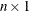vector of responses,is the overall mean,is the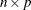matrix of standardized regressors, andis thevector of independent and identically distributed normal errors with mean 0 and unknown variance. The LASSO estimates of Tibshirani (1996) are the solution to the minimization problem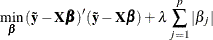for some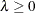, where.

Tibshirani (1996) suggested that the LASSO estimates can be interpreted as posterior mode estimates when the regression parameters have independent and identical Laplace priors. Park and Casella (2008) consider a fully Bayesian analysis by using a conditional Laplace prior specification of the formand the noninformative scale-invariant marginal prior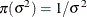on. Conditioning ofis important because it guarantees a unimodal full posterior. Park and Casella (2008) also note that any inverse-gamma prior formaintains conjugacy.

Exploiting the fact that the Laplace distribution can be represented as a scale mixture of normal densities with an exponential mixing density, Park and Casella (2008) propose the following hierarchical representation of the full model:The parametercan be given an independent, flat prior. After you integrate out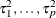, the conditional prior onhas the desired conditional Laplace distribution.

The Bayesian LASSO parametercan be chosen by using marginal maximum likelihood or an appropriate hyperprior. The example in the next section demonstrates the latter and considers, as suggested by Park and Casella (2008), the class of gamma priors on,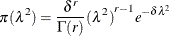## Example

This example from Park and Casella (2008) fits a Bayesian LASSO model to the diabetes data from Efron et al. (2004). In the original study, statisticians were asked to construct a model that predicted the response variable, Y, a quantitative measure of disease progression one year after baseline, from 10 covariates: Age, Sex, BMI, MAP, TC, LDL, HDL, TCH, LTG, and GLU. It was hoped that the model would produce accurate baseline predictions of response for future patients and that the form of the model would suggest which covariates were important factors in disease progression. The following SAS statements read the data and create the SAS data set Diabetes:


data diabetes;
input age sex bmi map tc ldl hdl tch ltg glu y;
sex=ifn(sex=2,1,0);
datalines;
59 2 32.1 101.00 157 93.2 38.0 4.00 4.8598 87.000 151
48 1 21.6 87.00 183 103.2 70.0 3.00 3.8918 69.000 75
72 2 30.5 93.00 156 93.6 41.0 4.00 4.6728 85.000 141

... more lines ...

60 2 24.9 99.67 162 106.6 43.0 3.77 4.1271 95.000 132
36 1 30.0 95.00 201 125.2 42.0 4.79 5.1299 85.000 220
36 1 19.6 71.00 250 133.2 97.0 3.00 4.5951 92.000 57
;


Before specifying the model in the MCMC procedure, you need to standardize the model’s regressors, excluding the indicator variable Sex. You can use the STDIZE procedure as follows to perform this task:


proc stdize data=diabetes out=std_diabetes;
var age bmi map tc ldl hdl tch ltg glu;
run;


The following statements specify the Bayesian LASSO in PROC MCMC:


ods graphics on;
ods output postintervals=intervals;
proc mcmc data=std_diabetes seed=45678 nmc=50000 propcov=quanew
monitor=(b0 beta1-beta10 tau1-tau10 sigma2 lasso)
outpost=posterior;

array D[10,10];
array beta beta1-beta10;
array mu0;
array data age sex bmi map tc ldl hdl tch ltg glu;

begincnst;
call identity(D);
call zeromatrix(mu0);
endcnst;

beginnodata;
lasso=sqrt(lambda);
b=lambda/2;

%macro loop;
%do k = 1 %to 10;
tau&k = exp(omega&k);
D[&k,&k]=sigma2*tau&k;
%end;
%mend loop;
%loop;
endnodata;

call mult(beta, data,xb);

parms lambda ;
prior lambda ~ gamma(1,scale=.1);
parms omega1-omega10;
prior omega: ~ expexpon(iscale=b);
parms sigma2 1;
prior sigma2 ~ igamma(shape = .1, iscale = .1);
parms b0 0;
prior b0 ~ general(0);
parms beta;
prior beta ~ mvn(mu0,D);

model y ~ normal(b0 + xb,var=sigma2);

run;


The ODS OUTPUT statement saves the posterior credible intervals in the SAS data set Intervals. The NMC= option in the PROC MCMC statement requests 50,000 MCMC iterations, excluding the burn-in iterations. A large sample is used because the posterior samples are highly autocorrelated. The PROPCOV= option in the PROC MCMC statement requests that the quasi-Newton method be used in constructing the initial covariance matrix for the Metropolis-Hastings algorithm. The OUTPOST= option saves the posterior samples in the data set Posterior.

The next four statements create arrays that are used in the model. The array D is the covariance matrix for the regression parameters Beta1Beta10. The array Beta is the vector of the regression parameters Beta1Beta10. The array Mu0 is the mean vector for the prior distribution of the regression parameters Beta1Beta10. The array Data is the matrix of regressors, excluding the intercept.

The BEGINCNST and ENDCNST statements define a statement block within which PROC MCMC processes the programming statements only during the setup stage of the simulation. You can use the BEGINCNST and ENDCNST statement block to initialize the matrices D and Mu0. D is initially set to an identity matrix, and Mu0 is initialized as a zero vector.

The BEGINNODATA and ENDNODATA statements define a block within which PROC MCMC processes the programming statements without stepping through the entire data set. The programming statements are executed only twice: at the first and last observations of the data set. Within this statement block, the parameters Lasso and b are defined. The macro %LOOP repopulates the matrix D. The purpose of the parameters Omega1Omega10 and their relationship with the parameters Tau1Tau10 are explained later.

The next statement uses the MULT CALL routine to define the matrix XB, which contains the product of the regressors and the regression parameters Beta1Beta10. That is, it contains the linear predictor, excluding the intercept.

The following block of statements declares the model parameters and assigns prior distribution to them. The parameter Lambda, which represents, is specified to have a gamma distribution. The parameters Omega1Omega10 are specified to have exponential exponential distributions. The parametershave exponential distributions, but modeling these parameters directly can cause convergence problems. Instead, the parameters Omega1Omega10 are modeled directly, and within the macro %LOOP the parameters Tau1Tau10, which represent, are defined as being the exponential of Omega1Omega10, respectively. The parameter Sigma2, which represents, is specified to have an inverse-gamma distribution. The parameter B0, which represent, is specified to have an improper uniform distribution. The parameter vector Beta, which represents, is specified to have a multivariate normal distribution with mean equal to 0 and variance matrix equal to D.

Finally, the MODEL statement specifies that the response variable Y have a normal distribution.

Output 1 shows that the Monte Carlo standard errors (MCSE) of each parameter are small relative to the posterior standard deviations (SD). A small MCSE/SD ratio indicates that the Markov chain has stabilized and that the mean estimates do not vary much over time.

Output 1: Monte Carlo Standard Errors

The MCMC Procedure

Monte Carlo Standard Errors
Parameter MCSE Standard
Deviation
MCSE/SD
b0 0.1105 3.7685 0.0293
beta1 0.0769 2.8187 0.0273
beta2 0.2072 5.8461 0.0354
beta3 0.1029 3.1602 0.0326
beta4 0.1093 3.3479 0.0326
beta5 0.3238 11.1592 0.0290
beta6 0.3241 11.4013 0.0284
beta7 0.1018 3.5868 0.0284
beta8 0.2234 7.4085 0.0302
beta9 0.1765 6.2293 0.0283
beta10 0.0992 3.3421 0.0297
tau1 0.0875 3.7978 0.0230
tau2 0.0925 4.0235 0.0230
tau3 0.1082 3.9813 0.0272
tau4 0.1124 3.8682 0.0291
tau5 0.0934 3.7605 0.0248
tau6 0.1107 3.8139 0.0290
tau7 0.0922 3.6053 0.0256
tau8 0.1004 3.6653 0.0274
tau9 0.1105 4.1032 0.0269
tau10 0.0969 3.7154 0.0261
sigma2 1.1459 202.7 0.00565
lasso 0.00400 0.1500 0.0266

Output 2 shows the Effective Sample Sizes table. The autocorrelation times for the parameters range from 1.59 to 62.83, and most of the efficiency rates are low. These results account for the relatively small effective sample sizes, given a nominal sample size of 50,000.

Output 2: Effective Sample Sizes

Effective Sample Sizes
Parameter ESS Autocorrelation
Time
Efficiency
b0 1164.0 42.9555 0.0233
beta1 1343.4 37.2178 0.0269
beta2 795.7 62.8345 0.0159
beta3 942.3 53.0600 0.0188
beta4 938.5 53.2742 0.0188
beta5 1188.0 42.0892 0.0238
beta6 1237.5 40.4033 0.0248
beta7 1241.1 40.2856 0.0248
beta8 1100.0 45.4557 0.0220
beta9 1245.4 40.1475 0.0249
beta10 1134.0 44.0920 0.0227
tau1 1883.1 26.5514 0.0377
tau2 1892.7 26.4173 0.0379
tau3 1353.3 36.9456 0.0271
tau4 1183.6 42.2445 0.0237
tau5 1619.6 30.8725 0.0324
tau6 1186.1 42.1539 0.0237
tau7 1529.5 32.6909 0.0306
tau8 1333.9 37.4850 0.0267
tau9 1378.2 36.2783 0.0276
tau10 1468.9 34.0388 0.0294
sigma2 31287.3 1.5981 0.6257
lasso 1409.3 35.4777 0.0282

The following SAS statements use the OUTPOST data set Posterior and the ODS OUTPUT data set Intervals to generate a table of the Bayesian LASSO parameter estimates, which are the modes of the posterior samples for B0 and Beta1Beta10, and their respective 95% HPD intervals:


proc means data=posterior mode;
var b0 beta1-beta10;
output out=parameters(drop=_TYPE_ _FREQ_) mode(b0 beta1-beta10)=b0 beta1-beta10;
run;

proc transpose data=parameters out=parameters;
run;


data parameters;
length parameter \$ 6;
set parameters(rename=(col1=mode _NAME_=Parameter));
label Parameter=;
index=_N_;
run;


proc sort data=parameters out=parameters;
by parameter;
run;

proc sort data=intervals out=intervals;
by parameter;
run;


data parameters(where=(~missing(mode)));
merge parameters intervals;
by parameter;
label parameter="Parameter" mode="Mode";
run;


proc sort data=parameters out=parameters;
by index;
run;


proc print data=parameters noobs label;
var parameter mode hpdlower hpdupper;
run;


Output 3 shows that the HPD intervals for the parameters Beta1, Beta5, Beta6, Beta8, and Beta10 all contain 0. Unlike what happens in the frequentist version of the LASSO, regression parameters are not set to 0, so the inclusion of 0 in the HPD interval is the only indication that a variable is a candidate for exclusion from the model. Based on this criterion, the variables Age, TC, LDL, TCH, and GLU are the leading candidates for exclusion from the model.

Output 3: Bayesian LASSO Parameter Estimates and 95% HPD Intervals

Parameter Mode HPDLower HPDUpper
b0 162.2 155.2 170.1
beta1 -1.4406 -5.8311 5.2856
beta2 -21.2417 -33.7811 -11.0211
beta3 26.5303 18.3849 30.6794
beta4 13.6935 9.4601 22.4973
beta5 -10.1466 -33.9135 9.7807
beta6 6.9991 -17.1198 27.7032
beta7 -13.3526 -17.2501 -3.3104
beta8 1.7061 -14.5072 14.3417
beta9 25.8343 15.8578 40.0418
beta10 0.3158 -2.5716 10.4408

## References

• Efron, B., Hastie, T. J., Johnstone, I. M., and Tibshirani, R. (2004), “Least Angle Regression (with Discussion),” Annals of Statistics, 32, 407–499.

• Kyung, M., Gill, J., Ghosh, M., and Casella, G. (2010), “Penalized Regression, Standard Errors, and Bayesian Lassos,” Bayesian Analysis, 5, 369–412.

• Park, T. and Casella, G. (2008), “The Bayesian Lasso,” Journal of the American Statistical Association, 103, 681–686.

• Tibshirani, R. (1996), “Regression Shrinkage and Selection via the Lasso,” Journal of the Royal Statistical Society, Series B, 58, 267–288.